Home > ACC7 > Chapter cc38 > Lesson cc38.3.1 > Problem8-133

8-133.
1. Solve each equation below for the given variable. Be sure to check your solution. Homework Help ✎

1. 6x − 11 = 3x + 16

2. −2(5 − 3x) + 5 = 9 + 3x

3.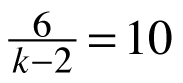4.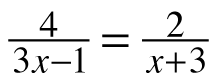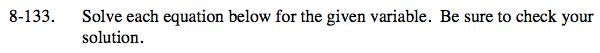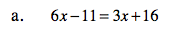Isolate x on one side and simplify.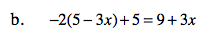Distribute −2 first, then isolate x and simplify.

−10 + 6x + 5 = 9 + 3x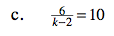How can you remove the fraction?

You can multiply both sides by k − 2.

$({\it k}-2)\frac{6}{{\it k}-2}=10({\it k} - 2)$

6 = 10(k − 2)
Now simplify.

6 = 10k − 20
26 = 10k

${\it k} = \frac{13}{5} = 2.6$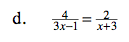How can you remove the fractions?

You can use cross multiplication.
4(x + 3) = 2(3x − 1)

x = 7
Remember to show your work.